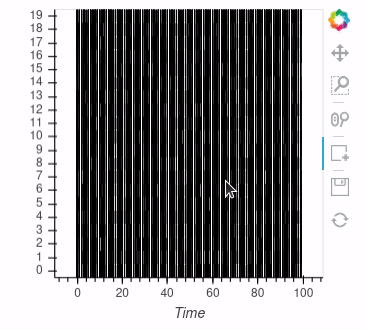# Using BoxEdit tool on hv.Spikes plot

Hello,

I’m trying to use the BoxEdit ROI drawing function on a plot composed of hv.Spike that have been subsampled, something like as with this question: https://discourse.holoviz.org/t/possible-to-use-decimate-on-hv-spikes-object/1003

Main Issue:
The BoxEdit drawing works on the plot before subsampling, but no longer works once the subsampling is applied.

Using modified code from the linked question:

``````raster = hv.NdOverlay({i: hv.Spikes(np.random.randint(0, 100, 1000), kdims='Time').opts(position=0.1*i)
for i in range(20)}).opts(yticks=[((i+1)*0.1-0.05, i) for i in range(20)]).opts(
opts.Spikes(spike_length=0.1),
opts.NdOverlay(show_legend=False))

def subsample(spikes, x_range, n=1000):
sliced = spikes.select(Time=x_range)
count = len(sliced)
if count <= n:
return sliced
return sliced.iloc[np.random.randint(0, count, n)]

x_range = hv.streams.RangeX(x_range=raster.range(1))
subsampled_raster = raster.apply(subsample, streams=[x_range])
``````

and formulating the BoxEdit function like this:

``````polys = hv.Polygons([])
box_stream = streams.BoxEdit(source=polys, num_objects=100)

polys = polys.opts(
opts.Polygons(active_tools=['box_edit'], fill_alpha=0.5))
``````

Prior to applying the subsample, using the BoxEdit works:

``````plot_all = raster * polys
plot_all.opts(show_legend=False)
``````But after applying, it no longer does:

``````x_range = hv.streams.RangeX(x_range=raster.range(1))
raster = raster.apply(subsample, streams=[x_range])

plot_sub = raster * polys
plot_sub.opts(show_legend=False)
``````

Any ideas on how to recover the BoxEdit functionality?
Thanks for any/all input!